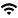# Department of Mathematics Calendar

Back to Listing

The Department of Mathematics Calendar has moved to Webtools. Anyone may submit an event by clicking the "+" button (upper right). Note: the Sponsor field is required. Just type "n/a"

All events will be reviewed before acceptance. Please email Shelby Koehne if you have any questions about submitting an event.

Note: you may search past and future events by clicking on the magnifying glass icon on the main Calendar page.

For an archive of past events: https://math.illinois.edu/research/seminars-department-calendar

## Number Theory Seminar: The polynomials X^2+(Y^2+1)^2 and X^2 + (Y^3+Z^3)^2 also capture their primes

Event Type
Seminar/Symposium
n/a
VirtualDate
Feb 22, 2022   11:00 am
Speaker
Jori Merikoski (Oxford)
Contact
Kevin Ford
E-Mail
ford126@illinois.edu
Views
12
`Abstract: We show that there are infinitely many primes of the form X^2+(Y^2+1)^2 and X^2 + (Y^3+Z^3)^2. `
`Our work builds on the famous Friedlander-Iwaniec result on primes of the form X^2+Y^4. `
`More precisely, Friedlander and Iwaniec obtained an asymptotic formula for the number of primes of this form. `
`For the argument we need to estimate Type II sums, which is achieved by an application of the Weil bound, `
`both for point-counting and for exponential sums over curves. The type II information we get is too narrow `
`for an asymptotic formula, but we can apply Harman's sieve method to establish a lower bound of the `
`correct order of magnitude for the number of primes of the form X^2+(Y^2+1)^2 and X^2 + (Y^3+Z^3)^2. `

Meeting ID: 840 2782 4197